## 二叉树的递归算法好难理解，求大神分享感悟

2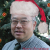递归(recursion) 又称递回，在数学与计算机科学中，是指在函数的定义中使用函数自身的方法。

一般来说，递归需要有边界条件、递归前进段和递归返回段。当边界条件不满足时，递归前进；当边界条件满足时，递归返回。

构成递归需具备的条件：
1. 子问题须与原始问题为同样的事，且更为简单；
2. 不能无限制地调用本身，须有个出口，化简为非递归状况处理。

代码取自美国课本 "Java How to Program "(Deitel & Detel)的练习： 20.25。

private void inorderHelper( TreeNode node ){
if ( node == null ) //若节点为空
return; //无任何操作

inorderHelper( node.leftNode ); //有序遍历下一级左子树
System.out.print( node.data + " " ); //输出节点数据
inorderHelper( node.rightNode );//有序遍历下一级右子树
}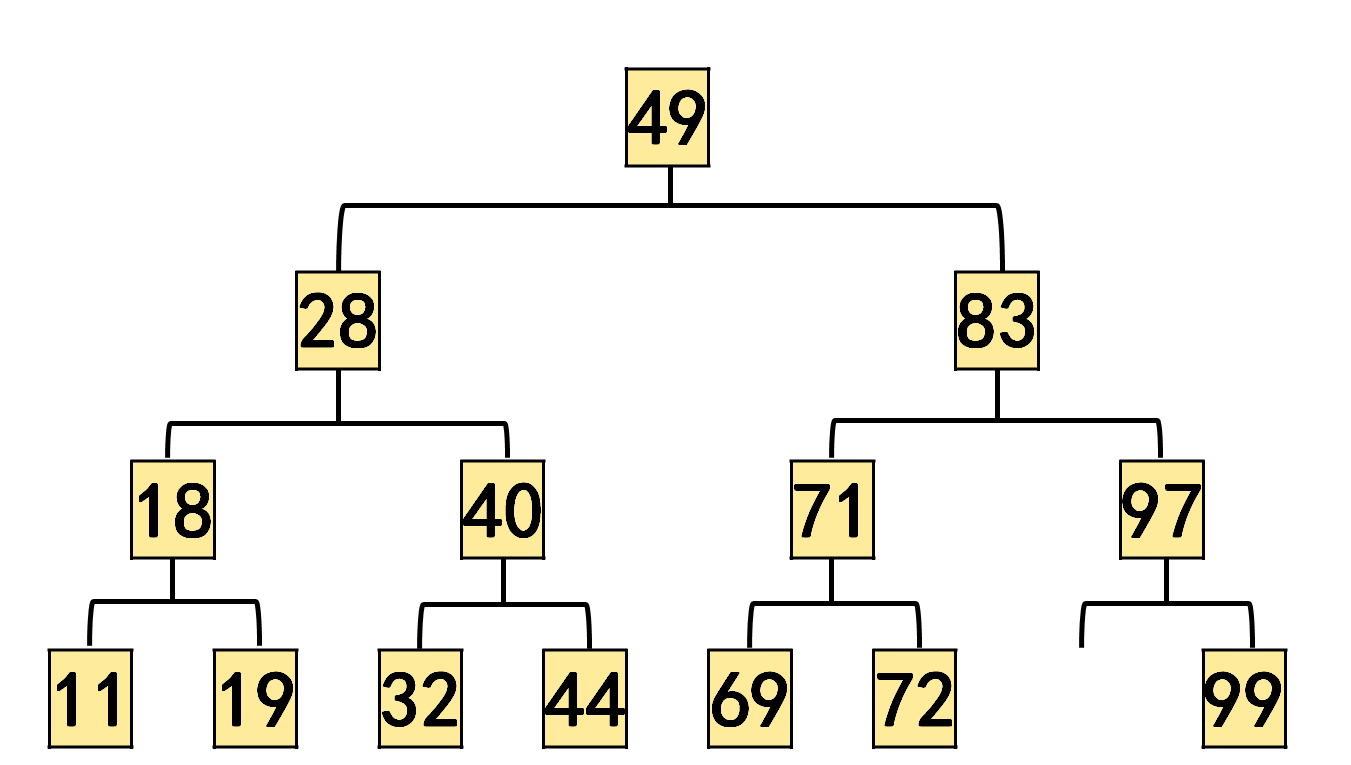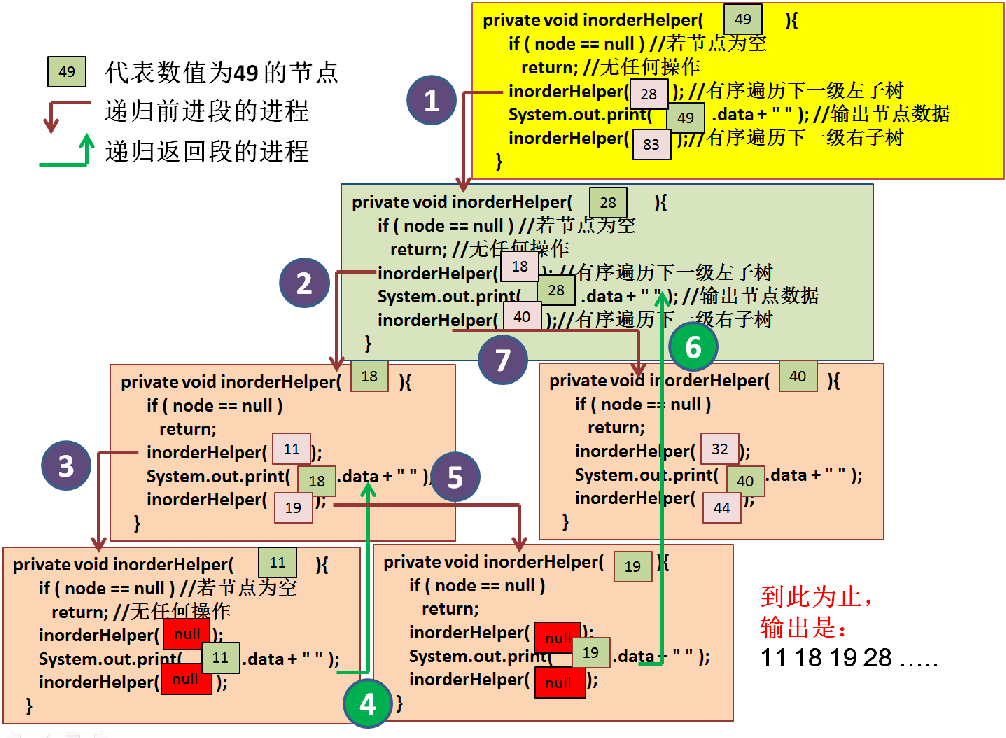1. 前进段的进程 1 ：鉴于以树根 节点 "49" 为参数，调用 inorderHelper(...)，开始调用以下一级树根 节点 "28" 为参数 的inorderHelper(...) 方法。
2. 前进段的进程 2 ：鉴于以树根 节点 "28" 为参数，调用 inorderHelper(...)，开始调用以下一级树根 节点 "18" 为参数 的 inorderHelper(...) 方法。
3. 前进段的进程 3 ：鉴于以树根 节点 "18" 为参数，调用 inorderHelper(...)，开始调用以下一级树根 节点 "11" 为参数 的 inorderHelper(...) 方法。
4. 节点 "11" 为叶节点，递归前进到终点。开始启动返回操作， 输出其数值 11。
5. 至此，参数为 节点 "11" 的 方法 inorderHelper(...) 执行完毕。返回进程 4 启动下一个 输出：18。
6. 输出 18 的代码行执行完毕，进入前进段进程 5， 执行接下来一行的代码：调用参数为 节点 "19" 的节点的方法 inorderHelper(...)
7. 节点 "19" 为叶节点，递归前进到终点。开始启动返回操作， 输出其数值 19。
8. 至此，参数为 节点 "19" 的 方法 inorderHelper(...) 执行完毕。返回进程 6 启动下一个 输出：28。
9. .....

``````// Deitel & Detel, 20.25
// class TreeNode definition
class TreeNode {

// public access members
public TreeNode leftNode;
public int data;
public TreeNode rightNode;

// initialize data and make this a leaf node
public TreeNode( int nodeData )
{
data = nodeData;
leftNode = rightNode = null;  // node has no children
}

// locate insertion point and insert new node; ignore duplicate values
public synchronized void insert( int insertValue )
{
// insert in left subtree
if ( insertValue < data ) {

// insert new TreeNode
if ( leftNode == null )
leftNode = new TreeNode( insertValue );

else // continue traversing left subtree
leftNode.insert( insertValue );
}

// insert in right subtree
else if ( insertValue > data ) {

// insert new TreeNode
if ( rightNode == null )
rightNode = new TreeNode( insertValue );

else // continue traversing right subtree
rightNode.insert( insertValue );
}

} // end method insert

// get right child
public synchronized TreeNode getRight()
{
return rightNode;
}

// get left child
public synchronized TreeNode getLeft()
{
return leftNode;
}

// return the data
public synchronized Object getData()
{
return new Integer( data );
}

}  // end class TreeNode

public class TreeTest {
private TreeNode root;

public TreeTest()
{
root = null;
}

// insert a new node in the binary search tree
public synchronized void insertNode( Integer value )
{
if ( root == null )
root = new TreeNode( value.intValue() );

else
root.insert( value.intValue() );
}

// begin preorder traversal
public synchronized void preorderTraversal()
{
preorderHelper( root );
}

// recursive method to perform preorder traversal
private void preorderHelper( TreeNode node )
{
if ( node == null )
return;

System.out.print( node.data + " " );
preorderHelper( node.leftNode );
preorderHelper( node.rightNode );
}

// begin inorder traversal
public synchronized void inorderTraversal()
{
inorderHelper( root );
}

// recursive method to perform inorder traversal
private void inorderHelper( TreeNode node )
{
if ( node == null )
return;

inorderHelper( node.leftNode );
System.out.print( node.data + " " );
inorderHelper( node.rightNode );
}

// begin postorder traversal
public synchronized void postorderTraversal()
{
postorderHelper( root );
}

// recursive method to perform postorder traversal
private void postorderHelper( TreeNode node )
{
if ( node == null )
return;

postorderHelper( node.leftNode );
postorderHelper( node.rightNode );
System.out.print( node.data + " " );
}

// begin printing tree
public void outputTree()
{
outputTreeHelper( root, 0 );
}

// recursive method to print tree
private void outputTreeHelper( TreeNode currentNode, int spaces )
{
// recursively print right branch, then left
if ( currentNode != null ) {
outputTreeHelper( currentNode.getRight(), spaces + 5 );

for ( int k = 1; k <= spaces; k++ )
System.out.print( " " );

System.out.println( currentNode.getData().toString() );
outputTreeHelper( currentNode.getLeft(), spaces + 5 );
}
}

public static void main( String args[] )
{
TreeTest tree = new TreeTest();
int intVal;

System.out.println( "Inserting the following values: " );
/*
// create Objects to store in tree
for ( int i = 1; i <= 10; i++ ) {
intVal = ( int ) ( Math.random() * 100 );
System.out.print( intVal + " " );
tree.insertNode( new Integer( intVal ) );
}
*/

tree.insertNode( new Integer( 49) );

tree.insertNode( new Integer( 28 ) );
tree.insertNode( new Integer( 83 ) );
tree.insertNode( new Integer( 18 ) );
tree.insertNode( new Integer( 19) );
tree.insertNode( new Integer( 11 ) );
tree.insertNode( new Integer( 40 ) );
tree.insertNode( new Integer( 32 ) );
tree.insertNode( new Integer( 44) );
tree.insertNode( new Integer( 71) );
tree.insertNode( new Integer( 72) );
tree.insertNode( new Integer( 69 ) );
tree.insertNode( new Integer( 97) );
tree.insertNode( new Integer( 99) );

// run three different traversal types
System.out.println ( "\n\nPreorder traversal" );
tree.preorderTraversal();

System.out.println ( "\n\nInorder traversal" );
tree.inorderTraversal();

System.out.println ( "\n\nPostorder traversal" );
tree.postorderTraversal();

// print a depiction of the tree
System.out.println( "\n\n" );
tree.outputTree();
}

}  // end class TreeTest
``````

0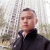0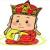1.无论B+还是二叉只要是树这种数据结构必然是由节点组成，即一定有NODE这种结构存在。

2.二叉树的NODE结构必然包含0-2三种情况之一：

1）NODE 为空，没有节点

2）NODE 只包含 一个节点 即LEFTNODE或RIGHTNODE，这里可以随意定哪个节点

3）NODE包含LEFTNODE和RIGHTNODE一个左一个右。综上所述如果存在node struct的话是这样的：

``````class Node{

public Node LeftNode;

public Node RightNode;

}``````

3.每个节点每个LEFTNODE或RIGHTNODE可能又包含0-2三个节点。而递归的意思是

4.递归的意思非常简单就是在函数的代码里调用这个函数。

5.树型结构使用递归的原因在于并不清楚子节点（LEFT或RIGHT)节点里是否包含子节点，当然

0

0#### 根据 动画：二叉树遍历的多种姿势，画出的 中序递归遍历的代码操作顺序

``````void inorderTraversal(Node node){
if (node) {
inorderTraversal(node.left);
System.out.println(node.data);
inorder Traversal(node.right);
}
}``````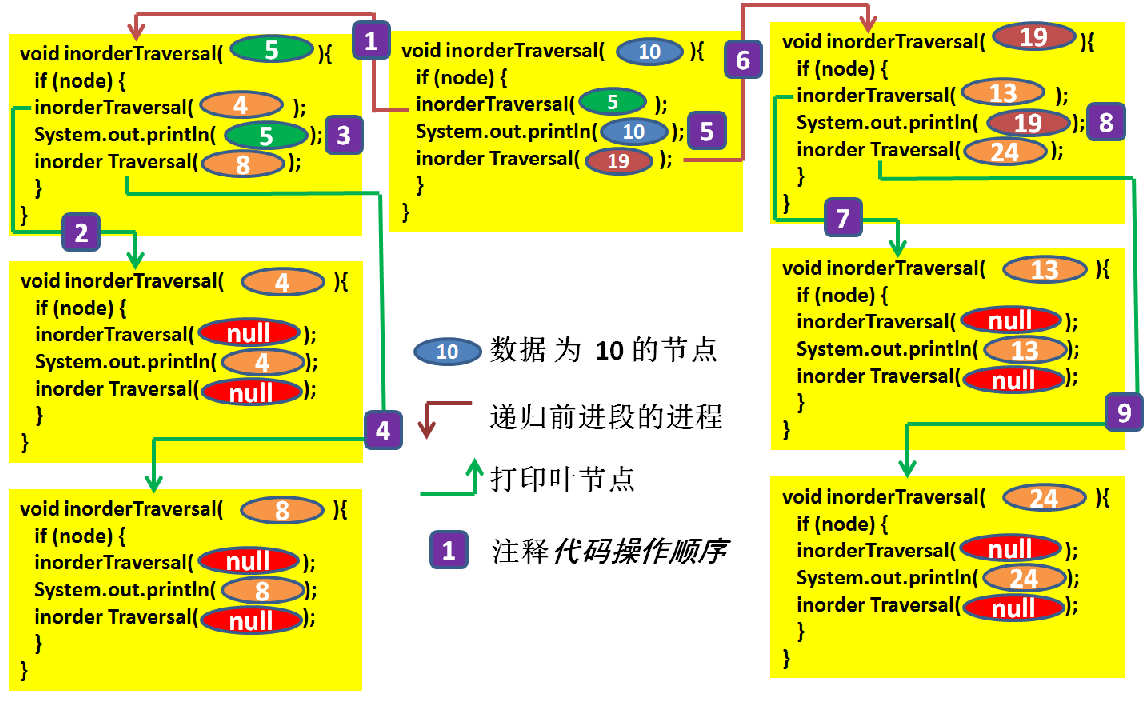1. 先遍历 总树根的左子树，左子树的树根是数值为 5 的节点。
2. 打印 数值为4的叶节点*。
3. 输出 数值为5的节点 (左子树的树根)。
4. 打印 数值为8的叶节点*。(至此，树根数值为 5 的节点的 左子树 遍历完毕)
5. 输出 数值为10的节点 (总树根)。
6. 然后 遍历 总树根的右子树，右子树的树根是数值为 19 的节点。
7. 打印 数值为13的叶节点*。
8. 输出 数值为19的节点(右子树的树根)。
9. 打印 数值为24的叶节点*。(至此，树根数值为 19 的节点的 右子树 遍历完毕。进而，总树根数值为10的节点的二叉查询树 遍历完成。)

* 调用 树根为 叶节点 的(子)树 的中序递归遍历方法 void inorderTraversal(Node root)，由于其左、右子树的树根都为空，故仅输出这个叶节点的数据数值。因此简称："打印叶节点"。先输出左子树，然后输出当前节点10，最后输出右子树； 对于其左子树来说，同样以这样的顺序，则先输出左子树4，然后输出节点值5，最后输出右子树8； 对于其右子树，同样以这样的顺序，则先,输出左子树13，然后输出节点值19，最后输出右子树24； 最终得到中序遍历输出为：4,5,8,10,13,19,24。